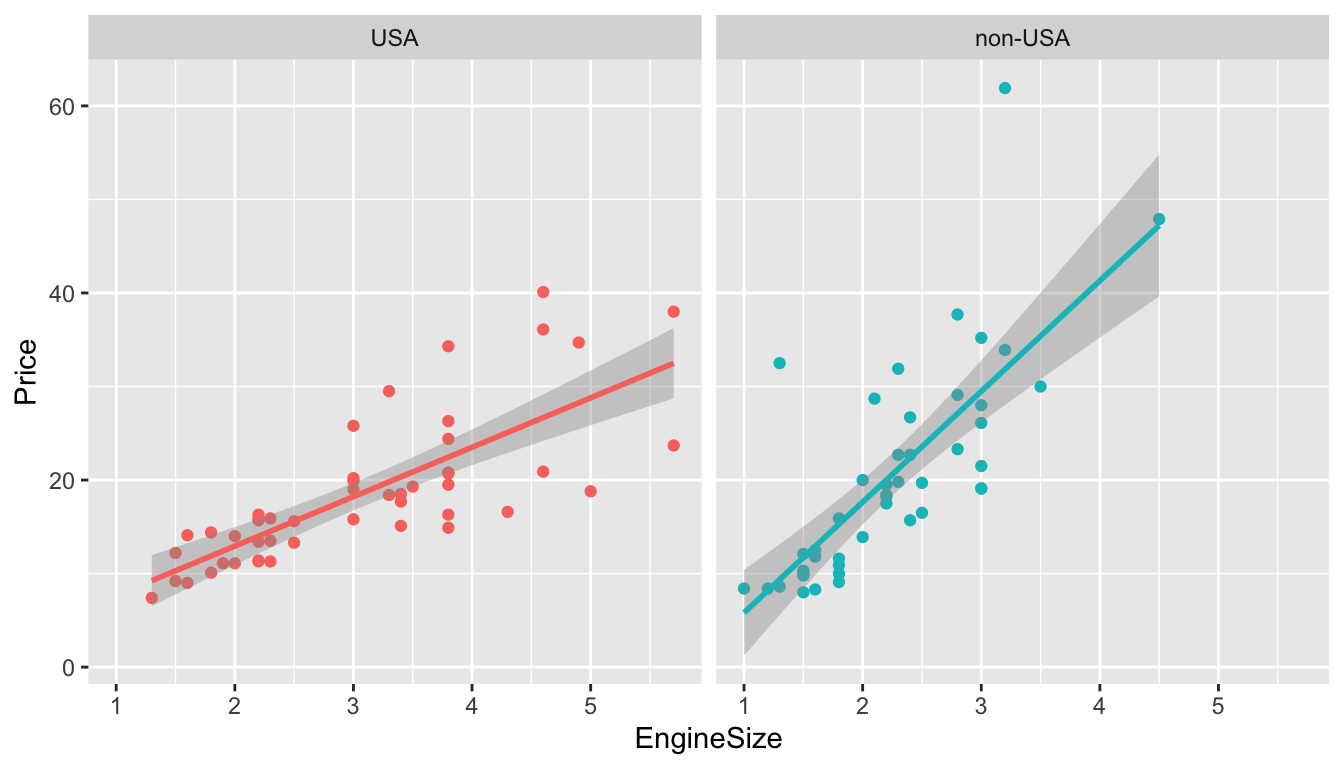##### Remember to change the author: field on this Rmd file to your own name.

library(tidyverse)
## ── Attaching packages ─────────────────────────────────────────────────── tidyverse 1.2.1 ──
## ✔ ggplot2 3.2.1     ✔ purrr   0.3.3
## ✔ tibble  2.1.3     ✔ dplyr   0.8.3
## ✔ tidyr   1.0.0     ✔ stringr 1.4.0
## ✔ readr   1.3.1     ✔ forcats 0.4.0
## ── Conflicts ────────────────────────────────────────────────────── tidyverse_conflicts() ──
## ✖ dplyr::lag()    masks stats::lag()
Cars93 <- as_tibble(MASS::Cars93)

### Testing 2 x 2 tables

Doll and Hill’s 1950 article studying the association between smoking and lung cancer contains one of the most important 2 x 2 tables in history.

Here’s their data:

smoking <- as.table(rbind(c(688, 650), c(21, 59)))
dimnames(smoking) <- list(has.smoked = c("yes", "no"),
lung.cancer = c("yes","no"))
smoking
##           lung.cancer
## has.smoked yes  no
##        yes 688 650
##        no   21  59

(a) Use fisher.test() to test if there’s an association between smoking and lung cancer.

# Edit me

(b) What is the odds ratio? Interpret this quantity.

# Edit me

(c) Are your findings statistically significant?

# Edit me

### Plotting error bars

Using Doll and Hill’s smoking data and, construct a bar graph with accompanying error bars showing the proportion of study participants with lung cancer.

To succeed in this exercise, you’ll have to follow along careful with the lecture notes. Please read the section titled “Plotting the table values with confidence”.

# Edit me

### ANOVA with birthwt data

Let’s form our favourite birthwt data set.

# Import data, rename variables, and recode factors all in one set of piped
# commands
birthwt <-  as_tibble(MASS::birthwt)  %>%
rename(birthwt.below.2500 = low,
mother.age = age,
mother.weight = lwt,
mother.smokes = smoke,
previous.prem.labor = ptl,
hypertension = ht,
uterine.irr = ui,
physician.visits = ftv,
birthwt.grams = bwt) %>%
mutate(race = recode_factor(race, 1 = "white", 2 = "black", 3 = "other")) %>%
mutate_at(c("mother.smokes", "hypertension", "uterine.irr", "birthwt.below.2500"),
~ recode_factor(.x, 0 = "no", 1 = "yes"))

(a) Create a new factor that categorizes the number of physician visits into four levels: 0, 1, 2, 3 or more.

# Edit me

Hint: One way of doing this is with recode by specifying .default = "3 or more". Have a look at the help file for recode to learn more.

(b) Run an ANOVA to determine whether the average birth weight varies across number of physician visits. Interpret the results.

# Edit me

### Linear regression with Cars93 data

Below is figure showing how Price varies with EngineSize in the Cars93, with accompanying regression lines. There are two plots, one for USA cars, and one for non-USA cars.

qplot(data = Cars93, x = EngineSize, y = Price, colour = Origin) +
facet_wrap("Origin") +
stat_smooth(method = "lm") +
theme(legend.position="none")(a) Use the lm() function to regress Price on EngineSize and Origin

# Edit me

(b) Run plot() on your lm object. Do you see any problems?

par(mfrow = c(2,2))
# Edit me

(c) Try running a linear regression with log(Price) as your outcome.

# Edit me

(d) Run plot() on your new lm object. Do you see any problems?

par(mfrow = c(2,2))
# Edit me